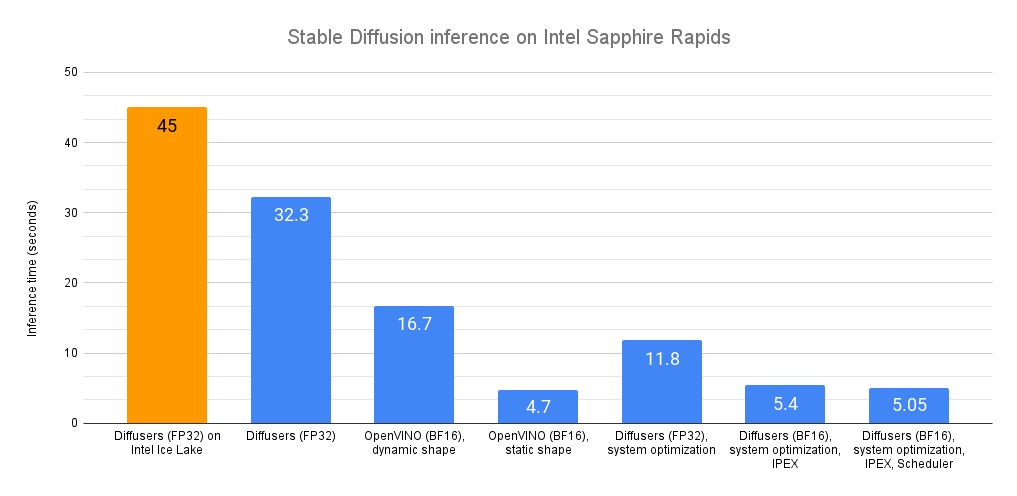# 在英特尔 CPU 上加速 Stable Diffusion 推理

## Diffusers 库

Diffusers 库使得用 Stable Diffusion 模型生成图像变得极其简单。如果你不熟悉 Stable Diffusion 模型，这里有一个很棒的 图文介绍

``````virtualenv sd_inference
source sd_inference/bin/activate
pip install transformers diffusers accelerate torch==1.13.1
``````

``````import time

def elapsed_time(pipeline, prompt, nb_pass=10, num_inference_steps=20):
# warmup
images = pipeline(prompt, num_inference_steps=10).images
start = time.time()
for _ in range(nb_pass):
_ = pipeline(prompt, num_inference_steps=num_inference_steps, output_type="np")
end = time.time()
return (end - start) / nb_pass
``````

``````from diffusers import StableDiffusionPipeline

model_id = "runwayml/stable-diffusion-v1-5"
pipe = StableDiffusionPipeline.from_pretrained(model_id)
prompt = "sailing ship in storm by Rembrandt"
latency = elapsed_time(pipe, prompt)
print(latency)
``````

## Optimum Intel 与 OpenVINO

Optimum Intel 用于在英特尔平台上加速 Hugging Face 的端到端流水线。它的 API 和 Diffusers 原始 API 极其相似，因此所需代码改动很小。

Optimum Intel 支持 OpenVINO，这是一个用于高性能推理的英特尔开源工具包。

Optimum Intel 和 OpenVINO 安装如下:

``````pip install optimum[openvino]
``````

``````from optimum.intel.openvino import OVStableDiffusionPipeline
...
ov_pipe = OVStableDiffusionPipeline.from_pretrained(model_id, export=True)
latency = elapsed_time(ov_pipe, prompt)
print(latency)

# Don't forget to save the exported model
ov_pipe.save_pretrained("./openvino")
``````

OpenVINO 会自动优化 `bfloat16` 模型，优化后的平均延迟下降到了 16.7 秒，相当不错的 2 倍加速。

``````ov_pipe.reshape(batch_size=1, height=512, width=512, num_images_per_prompt=1)
latency = elapsed_time(ov_pipe, prompt)
``````

## 系统级优化

jemalloctcmalloc 是两个很有意思的内存优化库。这里，我们使用 `jemalloc`，因为我们测试下来，它的性能比 `tcmalloc` 略好。 `jemalloc` 还可以用于针对特定工作负载进行调优，如最大化 CPU 利用率。详情可参考 `jemalloc`调优指南

``````sudo apt-get install -y libjemalloc-dev
``````

``````sudo apt-get install intel-mkl
``````

``````numactl -C 0-31 python sd_blog_1.py
``````

## IPEX 与 BF16

IPEX 扩展了 PyTorch 使之可以进一步充分利用英特尔 CPU 上的硬件加速功能，包括 AVX-512 、矢量神经网络指令 (Vector Neural Network Instructions，AVX512 VNNI) 以及 先进矩阵扩展 (AMX)。

``````pip install intel_extension_for_pytorch==1.13.100
``````

``````import torch
import intel_extension_for_pytorch as ipex
...
pipe = StableDiffusionPipeline.from_pretrained(model_id)

# to channels last
pipe.unet = pipe.unet.to(memory_format=torch.channels_last)
pipe.vae = pipe.vae.to(memory_format=torch.channels_last)
pipe.text_encoder = pipe.text_encoder.to(memory_format=torch.channels_last)
pipe.safety_checker = pipe.safety_checker.to(memory_format=torch.channels_last)

# Create random input to enable JIT compilation
sample = torch.randn(2,4,64,64)
timestep = torch.rand(1)*999
encoder_hidden_status = torch.randn(2,77,768)
input_example = (sample, timestep, encoder_hidden_status)

# optimize with IPEX
pipe.unet = ipex.optimize(pipe.unet.eval(), dtype=torch.bfloat16, inplace=True, sample_input=input_example)
pipe.vae = ipex.optimize(pipe.vae.eval(), dtype=torch.bfloat16, inplace=True)
pipe.text_encoder = ipex.optimize(pipe.text_encoder.eval(), dtype=torch.bfloat16, inplace=True)
pipe.safety_checker = ipex.optimize(pipe.safety_checker.eval(), dtype=torch.bfloat16, inplace=True)
``````

``````with torch.cpu.amp.autocast(enabled=True, dtype=torch.bfloat16):
latency = elapsed_time(pipe, prompt)
print(latency)
``````

## 调度器

Diffusers 库支持为每个 Stable Diffusion pipiline 配置 调度器 (scheduler)，用于在去噪速度和去噪质量之间找到最佳折衷。

``````from diffusers import StableDiffusionPipeline, DPMSolverMultistepScheduler
...
dpm = DPMSolverMultistepScheduler.from_pretrained(model_id, subfolder="scheduler")
pipe = StableDiffusionPipeline.from_pretrained(model_id, scheduler=dpm)
``````